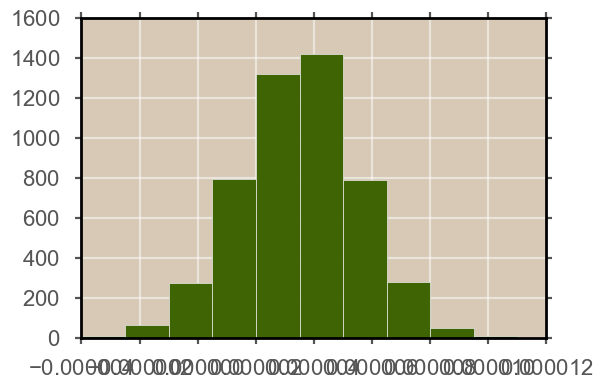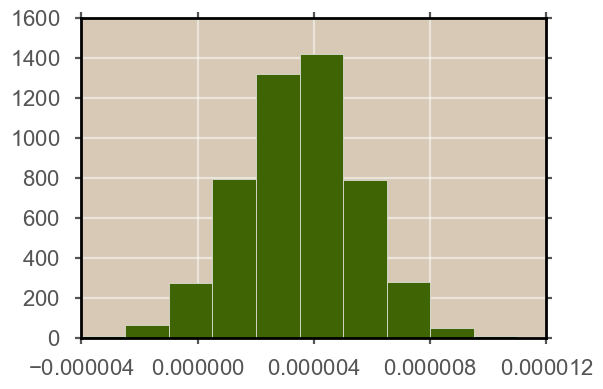A recent issue I had in my research is tick labels that overlap each other. It happens when there are too many leading zeros before the significant digit, yet too loo little for matplotlib to intelligently shift to scientific notation (about 4-7 leading zeroes). That result in the tick labels overlapping and making everything unreadable. It is not that much of a problem on exploratory code, but when I need a paper-worthy chart, it's an issue.

In :
import numpy as np
# import pandas as pd
import matplotlib.pyplot as plt
%matplotlib inline

In :
# Create random data of
x = np.random.normal(loc = 0.0000035, scale= 0.000002, size = 5000)
print (x)

[  3.84484418e-06   2.91685770e-06   3.88867504e-06 ...,   4.26532717e-06
3.18812399e-06   4.30168460e-06]


I have 5000 samples from the normal distribution. The samples have about 6 leading zeroes. Next, I'll create a histogram for the samples.

In :
fig, axs = plt.subplots(figsize=(6,4))
axs.hist(x)
plt.show()You can see how the labels of the x axis are overlapping and you can't understand any of them. I wanted a fast yet elegant way to deal with this. I didn't want to mess with rcParams for now (Although probably better in the long run).
.get_xticks() method returns a numpy array of all the labels. Now with the easy slicing mechanism of numpy arrays I can get all even places.
.set_xticks() method can set the new ticks to that value.

In :
fig, axs = plt.subplots(figsize=(6,4))
axs.hist(x)
axs.set_xticks(axs.get_xticks()[::2])
plt.show()There are better practices if you have many ticks on a many axes object, but this is a nice, elegant hack if you need it for a few paper-worthy charts, without the hassle.